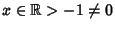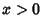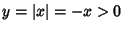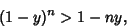## Bernoulli Inequality(1)

where,. This inequality can be proven by taking a Maclaurin Series of,(2)

Since the series terminates after a finite number of terms for Integral, the Bernoulli inequality foris obtained by truncating after the first-order term. When, slightly more finesse is needed. In this case, letso that, and take(3)

Since each Power ofmultiplies by a numberand since the Absolute Value of the Coefficient of each subsequent term is smaller than the last, it follows that the sum of the third order and subsequent terms is a Positive number. Therefore,(4)

or(5)

completing the proof of the Inequality over all ranges of parameters.